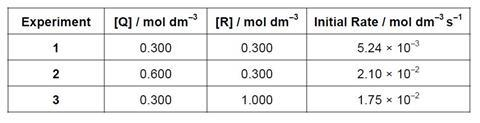Stop-watch at the ready, as students explore rate equation and clock reactions

A teacher asks the students to design a clock reaction to determine which is the rate determining step in the iodination of propanone.

## Pre-test questions

(Remember to give full references for any information beyond A-level that you find out)

1. Consider the reaction: 2 A + B → C + D
• Sketch a graph to show how the reaction rate (taken here as the change in [C] per unit time) would vary with [A] if the reaction was;
• I. zero order with respect to A
• II. first order with respect to A
• III. second order with respect to A
• A detailed study of the kinetics of the reaction revealed that the mechanism involved the formation of an intermediate E;
• Step one: A + A → C + E slow
• Step two: E + B → D fast

Which step is the rate determining step in the reaction?

1. The rate of a reaction can be determined experimentally using an initial rate method. This involves measuring the time it takes for some easily recognisable event to occur very early on in a reaction (usually when less than 10% of the reaction has occurred).
• For each of the following reactions, identify a visual change that you could measure the time taken to occur and hence used to determine the initial rate of the reaction;
• I. Mg + 2 HCl → MgCl2 + H2
• II. Na2 S2 O3 + 2 HCl → 2 NaCl + S + SO2 + H2 O
• III. CH3 CH2 Cl + OH→ CH3 CH2 OH + Cl
• In each of these reactions, the reaction time for the observed change is measured. Define the term “reaction rate” and hence explain how the reaction time can be converted into a reaction rate in any one of the reactions above.
1. The reaction Q + R P was studied using the method of initial rates. The initial rate of formation of was measured in three different experiments. The data are provided below• Use the data above to determine the rate equation for the reaction.
• What is the value of the rate constant, k?

## Equipment

### Chemicals

• Propanone solution [Highly flammable; Irritant], 100 cm3 of 2.0 M
• Hydrochloric acid solution [Low hazard], 100 cm3 of 1.0 M
• Iodine solution in aqueous potassium iodide [Low hazard], 30 cm3 of 0.005 M
• Distilled water

### Apparatus

• Burettes, burette stands and clamps and funnels (1 for each solution and water) (these can be shared between groups if needed), x 4
• Conical flask, 100 cm3, x 2
• Test tubes (for storage of acid and iodine solutions before addition)
• Stopwatch
• White tile or white paper x 2

## Health, safety and technical notes

• Read our standard health and safety guidance
• Wear eye protection
• Wear clothing protection, if desired.
• Propanone solution [Highly flammable; Irritant] see CLEAPSS Hazcard HC085a
• Hydrochloric acid solution [Low hazard] see CLEAPSS Hazcard HC047a
• Iodine solution in aqueous potassium iodide see CLEAPSS Hazcard HC047b
• The product of the reaction, iodopropanone, is a lachrymator (strongly irritant to eyes). The reaction mixture must therefore be disposed of as soon as measurements have been taken by flushing down a fume cupboard sink with lots of running water.